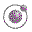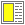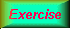Name binding, Recursion, Iteration, and Continuations - slide 21 : 42

Example of recursion: number-interval
The function number-interval returns a list of integers from a lower bound to an upper bound
```(define (number-interval f t)
(if (<= f t)
(cons f (number-interval (+ f 1) t))
'()))```The function number-interval-iter is an iterative, tail recursive variant of number-interval.A sample dialogue with the number interval functions.The append functionA list replication function